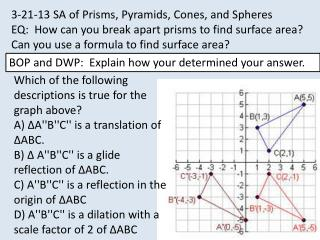Download Presentation3-21-13 SA of Prisms, Pyramids, Cones, and Spheres

# 3-21-13 SA of Prisms, Pyramids, Cones, and Spheres - PowerPoint PPT Presentation

3-21-13 SA of Prisms, Pyramids, Cones, and Spheres EQ: How can you break apart prisms to find surface area? Can you use a formula to find surface area? . BOP and DWP: Explain how your determined your answer. . Which of the following descriptions is true for the graph above?I am the owner, or an agent authorized to act on behalf of the owner, of the copyrighted work described.
Download Presentation## 3-21-13 SA of Prisms, Pyramids, Cones, and Spheres

An Image/Link below is provided (as is) to download presentation

Download Policy: Content on the Website is provided to you AS IS for your information and personal use and may not be sold / licensed / shared on other websites without getting consent from its author.While downloading, if for some reason you are not able to download a presentation, the publisher may have deleted the file from their server.

- - - - - - - - - - - - - - - - - - - - - - - - - - E N D - - - - - - - - - - - - - - - - - - - - - - - - - -
Presentation Transcript
1. 3-21-13 SA of Prisms, Pyramids, Cones, and Spheres EQ: How can you break apart prisms to find surface area? Can you use a formula to find surface area? BOP and DWP: Explain how your determined your answer. Which of the following descriptions is true for the graph above? A) ∆A''B''C'' is a translation of ∆ABC. B) ∆ A''B''C'' is a glide reflection of ∆ABC. C) A''B''C'' is a reflection in the origin of ∆ABC D) A''B''C'' is a dilation with a scale factor of 2 of ∆ABC

2. B) ∆ A''B''C'' is a glide reflection of ∆ABC

3. 2 3 1 2 3 4 2 5 1 6 1 3 5 8 1 7 Surface Area: Pyramids, Cones, and Spheres PRE-ALGEBRA LESSON 10-6 Use the Order of Operations to simplify each expression. 1. (9 ) + (8 ) 2. (12 ) + (15 ) 3. (24 ) + (3 ) 4. (32 ) + (14 ) 10-6

4. 1 21 PRE-ALGEBRA LESSON 10-6 Surface Area: Pyramids, Cones, and Spheres Solutions 1. • 93 + • 842. • 123 + • 153 = 6 + 4 = 9 + 6 = 10 = 15 3. • 244 + • 314. • 324 + • 142 = 4 + = 20 + 2 = 5 = 22 2 31 3 41 2 51 1 61 1 71 1 31 5 81 10-6

5. Check homework workbook pages 172, 173, 174

6. Finish you table started yesterday.

7. The fold crease Foldable Make a foldable for formulas for prisms and cylinders. Keep this in your notebook for reference. FORMULAS

8. FORMULAS The fold crease Foldable Fold over the top cut section and label each section on the outside. Do the same thing for the other shapes. Reopen the fold Rectangular Prism 6. Reopen the fold.

9. FORMULAS The fold crease Foldable Reopen the fold. *Draw each shape *Write the general formula for surface area of a rectangular prism. *Write the general formula for volume of A rectangular prism Rectangular Prism Triangular Prism Square Pyramid Cylinder cone

10. RECTANGULAR PRISM What are the steps to finding the surface area of a rectangular prism? Volume? SA = ph + 2B Remember the smiley face method Volume = Bh = lwh *base is a rectangle

11. CYLINDER 10 Diameter = 4 Volume = Bh = πr2h B =area of the base Surface Area = ph + 2B =πdh + πr2 B = area of the base

12. How do you find the volume of a triangular prism? Surface area? SA = ph + 2B (s+s+s)h + 2(bh) 2 *Base is a triangle Identify the height of the prism. V= Bh =(bh)H 2

13. Triangular Prism A prism whose base is a triangle Height Height

14. NET of a Triangular Prism Perimeter = Side + side + side Height of triangle Height Of prism Base is a triangle

15. How do you find the Surface Area and Volume of Triangular Prisms? Prisms have rectangular Faces. Height is measured from The base of the prism The BASE of a triangular prism a triangle.

16. SQUARE PYRAMID

17. NET of a square pyramid

18. Sketch the square pyramid in your notes.

19. Step 1L.A. = p Use the formula for lateral area. = • 20 • 8 = 80p = 4(5) and = 8. 1 2 1 2 PRE-ALGEBRA LESSON 10-6 Surface Area: Pyramids, Cones, and Spheres Find the surface area of the square pyramid. Step 2 S.A. = L.A. + B = 80 + 52 Lateral area = 80 and B = 52. = 80 + 25 = 105 The surface area of the pyramid is 105 m2. 10-6

20. Summary: Answer today’s essential questions. Homework: Handout on triangular prisms. Find the surface area and volume.